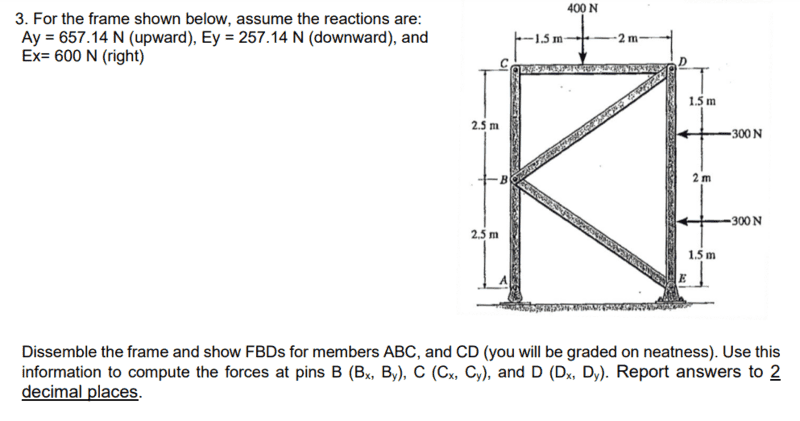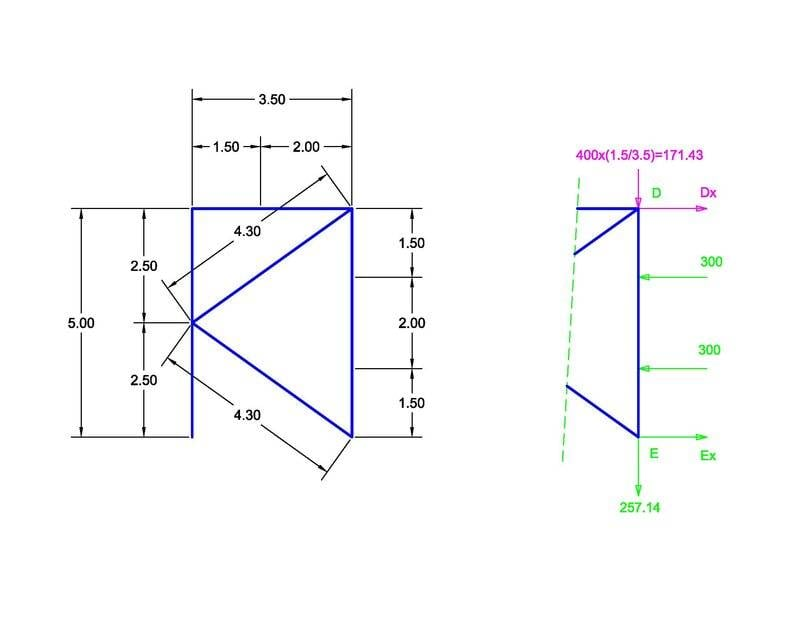# Frame Statics Problem

• Engineering
bob1352
Homework Statement:
This is a weekly assignment problem.
Relevant Equations:
Fnetx = 0
Fnety = 0
Sum of Moments at a point = 0
So for this problem I have already solved for the Y forces: Dy = 171.43 N, Cy = 228.57 N, and By = -428.57 N. For the X forces I split up the frame and took the moment of DE.

Me = 1.5(300) + 3.5(300) - 5(Dx), Dx = 300

For CD Dx = -Cx fo I got Cx = 300, as when you forces are two member Dx = -300 on member CD. Then I used the moment of ABC to solve for Bx

Ma = -2.5(Bx) + (5)300, Bx = 600 N.

I am almost postive my Y forces are correct, but I am unsure of the X-forces and I can balance the moments, but not the sum of the forces. Am I correct or am I missing something?Homework Helper
Gold Member
Note that support A is of a type that is unable to take horizontal loads.
Link BD is working under compression, while link BE is working under tension.
Therefore, there are two x-component forces acting on B in opposite directions.

bob1352
So Bx, Cx and Dx should be zero then? I am not using the method of joints, but the technique for multi-force members.

Homework Helper
Gold Member
So Bx, Cx and Dx should be zero then?
What makes you to think that way?
I am not using the method of joints, but the technique for multi-force members.
Could you show us the FBD that you have done so far?

bob1352
What makes you to think that way?

Could you show us the FBD that you have done so far?
Sure, here you go. I split the frame into parts as what the method of sections suggests.

#### Attachments

• Handwritten_2023-02-28_184521.pdf
765.2 KB · Views: 6
Homework Helper
Gold Member
Sure, here you go. I split the frame into parts as what the method of sections suggests.
Member DE acts as a beam supported at both ends, not like a cantilever beam.
That is because pivot E can't provide any reactive moment.

Simultaneously, pin D is not free to rotate around pivot E under the two lateral loads.
A force must be preventing that rotation from happening.
That force reaches pin D via ground-member AB-pin B-member BD.#### Attachments

• Frame Statics Problem (1).pdf
23.8 KB · Views: 7
Last edited: